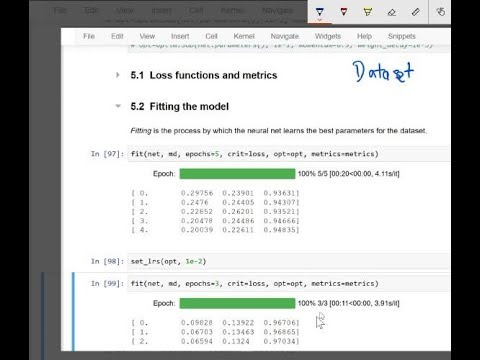Machine Learning 1: Lesson 10In today's lesson we'll further develop our NLP model by combining the strengths of naive bayes and logistic regression together, creating the hybrid "NB-SVM" model, which is a very strong baseline fo...
In today's lesson we'll further develop our NLP model by combining the strengths of naive bayes and logistic regression together, creating the hybrid "NB-SVM" model, which is a very strong baseline for text classification.

To do this, we'll create a new `nn.Module` class in pytorch, and look at what it's doing behind the scenes.

In the second half of the lesson we'll start our study of tabular and relational data using deep learning, by looking at the "Rossmann" Kaggle competition dataset. Today, we'll start down the feature engineering path on this interesting dataset.

We'll look at continuous vs categorical variables, and what kinds of feature engineering can be done for each, with a particular focus on using embedding matrices for categorical variables.